# Solutions of Electricity (Page No- 11) - Physics By Lakhmir Singh, Class 10 Class 10 Notes | EduRev

## Class 10 : Solutions of Electricity (Page No- 11) - Physics By Lakhmir Singh, Class 10 Class 10 Notes | EduRev

The document Solutions of Electricity (Page No- 11) - Physics By Lakhmir Singh, Class 10 Class 10 Notes | EduRev is a part of the Class 10 Course Class 10 Physics Solutions By Lakhmir Singh & Manjit Kaur.
All you need of Class 10 at this link: Class 10

Page No:11

Question 1:
By what name is the physical quantity coulomb/second called ?
Solution :
Ampere

Question 2:
What is the flow of charge called ?
Solution :
Electric Current.

Question 3:
What actually travels through the wires when you switch on a light ?
Solution :
Electrons.

Question 4:
Which particles constitute the electric current in a metallic conductor ?
Solution :
Electrons.

Question 5:
(a) In which direction does conventional current flow around a circuit ?
(b) In which direction do electrons flow ?

Solution :
(a) Conventional current flows from positive terminal of a battery to the negative terminal, through the outer circuit.
(b) Electrons flow from negative terminal to positive terminal of the battery (opposite to the direction of conventional current).

Question 6:
Which of the following equation shows the correct relationship between electrical units ?
1 A = 1 C/s or 1 C = 1 A/s
Solution :
1A = 1C/s

Question 7:
What is the unit of electric current ?
Solution :
Ampere.

Question 8:
(a) How many milliamperes are there in 1 ampere ?
(b) How many microamperes are there in 1 ampere ?

Solution :
(a) 1 amp = 103 milli amp.
(b) 1 amp = 106 micro amp.

Question 9:
Which of the two is connected in series : ammeter or voltmeter ?
Solution :
Ammeter is connected in series.

Question 10:
Compare how an ammeter and a voltmeter are connected in a circuit.
Solution :
Ammeter is connected in series in a circuit whereas voltmeter is connected in parallel.

Question 11:
What do the following symbols mean in circuit diagrams ?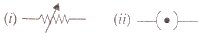Solution :
(i) Variable resistance.
(ii) A closed plug key.

Question 12:
If 20 C of charge pass a point in a circuit in 1 s, what current is flowing ?
Solution :
Given, Q = 20 C, t = 1s
I = ?
We know that:
I = Q/t.
I = 20/1 = 20A.

Question 13:
A current of 4 A flows around a circuit for 10 s. How much charge flows past a point in the circuit in this time ?
Solution :
Given, I = 4amp, C, t = 10s Q = ?
We know that:
I = Q/t.
Q = 4*10 = 40C.

Question 14:
What is the current in a circuit if the charge passing each point is 20 C in 40 s ?
Solution :
Given, Q = 20 C, t=1s
I = ?
We know that:
I = Q/t.
Thus I = 20/40 = 0.5A.

Question 15:
Fill in the following blanks with suitable words :
(a) A current is a flow of……….. For this to happen there must be a………….
(b) Current is measured in……… using an………… placed in……… in a circuit.

Solution :
(a) electrons; closed
(b) amperes; ammeter; series.

Question 16:
(a) Name a device which helps to maintain potential difference across a conductor (say, a bulb).
If a potential difference of 10 V causes a current of 2 A to flow for 1 minute, how much energy is
transferred ?

Solution :
(a) Cell or battery helps to maintain potential difference across a conductor.
(b) Given: p.d. = 10 V, I = 2amp, t = 1 min = 60s.
We know that:
I=Q/t.
Thus, Q=Ixt.
Q = 2×60.
Q = 120 C.
Work done = p.d. x charge moved
Work done = 120x10J
Work done = 1200J.

Question 17:
(a) What is an electric current ? What makes an electric current flow in a wire ?
(b) Define the unit of electric current (or Define ampere).

Solution :
(a) An electric currrent is a flow of electric charges (electrons) through a conductor.
Potential difference between the ends of the wire makes electric current to flow in the wire.
(b) When 1 coulomb of charge flows through any cross-section of a conductor in 1 second, the electric current flowing through it is said to be 1 ampere.

Question 18:
What is an ammeter ? How is it connected in a circuit ? Draw a diagram to illustrate your answer.
Solution :
Ammeter is a device used for the measurement of electric current. It is always connected in series with the circuit in which the current is to be measured.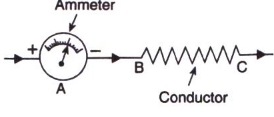Question 19:
(a) Write down the formula which relates electric charge, time and electric current.
(b) A radio set draws a current of 0.36 A for 15 minutes. Calculate the amount of electric charge that flows through the circuit.

Solution :
(a). Work done = Potential difference x charge moved.
(b). I = 0.36A, t = 15min = 900seconds.
Q = Ixt
= 0.36×900
= 324C.

Question 20:
Why should the resistance of :
(a) an ammeter be very small ?
(b) a voltmeter be very large ?

Solution :
(a) The resistance of an ammeter should be very small so that it may not change the value of the current flowing in the circuit.
(b) The resistance of a voltmeter should be very large so that it takes a negligible current from the current.

Question 21:
Draw circuit symbols for (a) fixed resistance (b) variable resistance (c) a cell (d) a battery of three cells (e) an open switch (f) a closed switch.
Solution :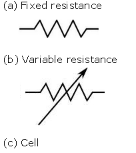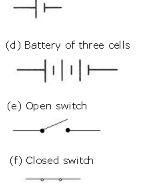Question 22:
What is a circuit diagram ? Draw the labelled diagram of an electric circuit comprising of a cell, a resistor, an ammeter, a voltmeter and a closed switch (or closed plug key). Which of the two has a large resistance : an ammeter or a voltmeter ?
Solution :
A diagram which indicates how different components in a circuit have been connected by using the electrical symbols for the components is called a circuit diagram.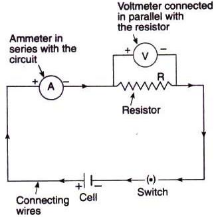A voltmeter has a large resistance.

Question 23:
If the charge on an electron is 1.6 x 10-19 coulombs, how many electrons should pass through a conductor in 1 second to constitute 1 ampere current ?
Solution :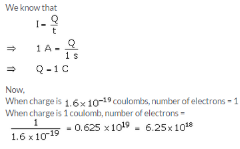Question 24:
The p.d. across a lamp is 12 V. How many joules of electrical energy are changed into heat and light when :
(a) a charge of 1 C passes through it ?
(b) a charge of 5 C passes through it ?
(c) a current of 2 A flows through it for 10 s ?
Solution :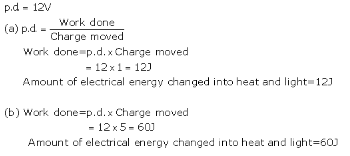(c) I = Q/t

Q= I x t

= 2 x 10 = 20c

Work done = p.d x Charge moved

= 12 x 20 = 2400

Amount of electrical energy changed into heat and light = 2400

Offer running on EduRev: Apply code STAYHOME200 to get INR 200 off on our premium plan EduRev Infinity!

94 docs

,

,

,

,

,

,

,

,

,

,

,

,

,

,

,

,

,

,

,

,

,

,

,

,

;